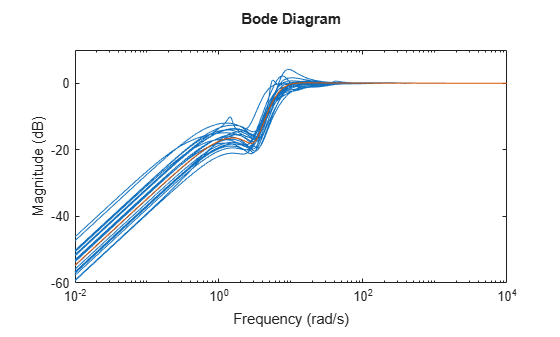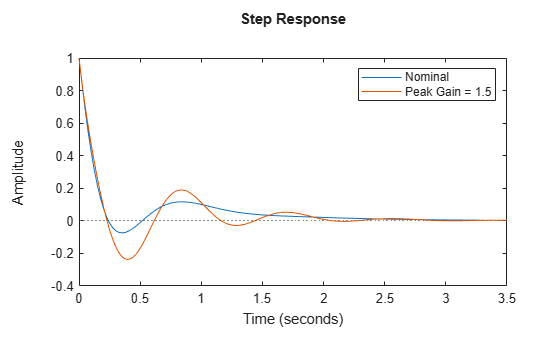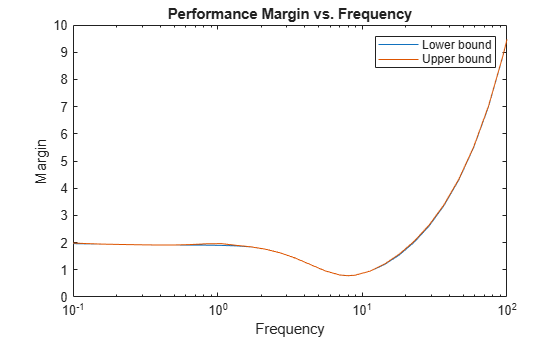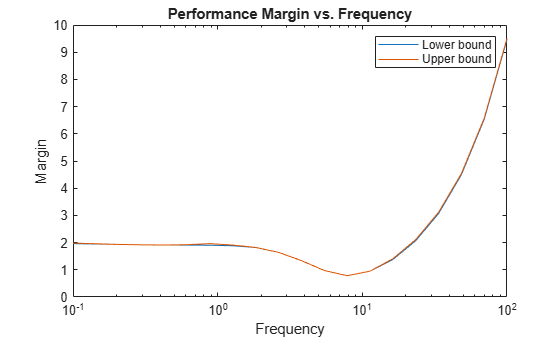Main Content

# robgain

Robust performance of uncertain system

## Syntax

``````[perfmarg,wcu] = robgain(usys,gamma)``````
``````[perfmarg,wcu] = robgain(usys,gamma,w)``````
``````[perfmarg,wcu] = robgain(___,opts)``````
``````[perfmarg,wcu,info] = robgain(___)``````

## Description

example

``````[perfmarg,wcu] = robgain(usys,gamma)``` calculates the robust performance margin for an uncertain system and the performance level `gamma`. The performance of `usys` is measured by its peak gain or peak singular value (see Robustness and Worst-Case Analysis). The performance margin is relative to the uncertainty level specified in `usys`. A margin greater than 1 means that the gain of `usys` remains below `gamma` for all values of the uncertainty modeled in `usys`. A margin less than 1 means that at some frequency, the gain of `usys` exceeds `gamma` for some values of the uncertain elements within their specified ranges. For example, a margin of 0.5 implies the following: The gain of `usys` remains below `gamma` as long as the uncertain element values stay within 0.5 normalized units of their nominal values.There is a perturbation of size 0.5 normalized units that drives the peak gain to the level `gamma`.The structure `perfmarg` contains upper and lower bounds on the actual performance margin and the critical frequency at which the margin upper bound is smallest. The structure `wcu` contains the uncertain-element values that drive the peak gain to the level `gamma`.```

example

``````[perfmarg,wcu] = robgain(usys,gamma,w)``` assesses the robust performance margin for the frequencies specified by `w`.If `w` is a cell array of the form `{wmin,wmax}`, then `robgain` restricts the performance margin computation to the interval between `wmin` and `wmax`.If `w` is a vector of frequencies, then `robgain` computes the performance margin at the specified frequencies only.```

example

``````[perfmarg,wcu] = robgain(___,opts)``` specifies additional options for the computation. Use `robOptions` to create `opts`. You can use this syntax with any of the previous input-argument combinations.```
``````[perfmarg,wcu,info] = robgain(___)``` returns a structure with additional information about the performance margins and the perturbations that drive the gain to `gamma`. See `info` for details about this structure. You can use this syntax with any of the previous input-argument combinations.```

## Examples

collapse all

Consider a control system whose plant contains both parametric uncertainty and dynamic uncertainty. Create a model of the plant using uncertain elements.

```k = ureal('k',10,'Percent',40); delta = ultidyn('delta',[1 1]); G = tf(18,[1 1.8 k]) * (1 + 0.5*delta);```

Create a model of the controller, and build the closed-loop sensitivity function, `S`. The sensitivity measures the closed-loop response at the plant output to a disturbance at the plant input.

```C = pid(2.3,3,0.38,0.001); S = feedback(1,G*C); bodemag(S,S.NominalValue)```The peak gain of the nominal response is very nearly 1, but some of the sampled systems within the uncertainty range exceed that level. Suppose that you can tolerate some ringdown in the response but do not want the peak gain to exceed 1.5. Use `robgain` to find out how much uncertainty the system can have while the peak gain remains below 1.5.

```[perfmarg,wcu] = robgain(S,1.5); perfmarg```
```perfmarg = struct with fields: LowerBound: 0.7821 UpperBound: 0.7837 CriticalFrequency: 7.8565 ```

The `LowerBound` and `UpperBound` fields of `perfmarg` show that the robust performance margin is around 0.78. This result means that there is a perturbation of only about 78% of the uncertainty specified in S with peak gain exceeding 1.5.

You can use `uscale` to examine what that normalized uncertainty of 78% means in terms of actual ranges of uncertainty. Scale all the uncertain elements in `S` to create a model of the closed-loop system with the maximum level of uncertainty that meets the performance requirement.

```factor = perfmarg.LowerBound; S_scaled = uscale(S,factor)```
```S_scaled = Uncertain continuous-time state-space model with 1 outputs, 1 inputs, 4 states. The model uncertainty consists of the following blocks: delta: Uncertain 1x1 LTI, peak gain = 0.782, 1 occurrences k: Uncertain real, nominal = 10, variability = [-31.3,31.3]%, 1 occurrences Type "S_scaled.NominalValue" to see the nominal value, "get(S_scaled)" to see all properties, and "S_scaled.Uncertainty" to interact with the uncertain elements. ```

The display shows how the uncertain elements in `S_scaled` have changed: the peak gain of the `ultidyn` element `delta` is reduced from 1 to 0.78, and the range of variation of the uncertain real parameter `k` is reduced from ±40% to ±31.3%.

The output `wcu` is a structure that contains the perturbations to `k` and `delta` that correspond to the target maximum performance of 1.5. Verify that the values in `wcu` cause `Smax` to achieve the gain level of 1.5 by substituting them into `S`.

```Smax = usubs(S,wcu); getPeakGain(Smax,1e-6)```
```ans = 1.5001 ```

Examine the disturbance rejection of the system with these values.

```step(S.NominalValue,Smax) legend('Nominal','Peak Gain = 1.5')```The `CriticalFrequency` field of `perfmarg` contains the frequency at which the peak gain reaches 1.5.

Examine the relative sensitivity of the robust performance margin to the uncertain elements of the system. Consider a model of a control system containing uncertain elements.

```k = ureal('k',10,'Percent',50); delta = ultidyn('delta',[1 1]); G = tf(18,[1 1.8 k]) * (1 + 0.15*delta); C = pid(2.3,3,0.38,0.001); S = feedback(1,G*C);```

Create an options set for `robgain` that enables the sensitivity calculation.

`opts = robOptions('Sensitivity','On');`

Calculate the robust performance margin of the system relative to a peak gain of 1.5, specifying the `info` output to access additional information about the calculation.

`[perfmarg,wcu,info] = robgain(S,1.5,opts);`

Examine the `Sensitivity` field of `info`.

`info.Sensitivity`
```ans = struct with fields: delta: 75 k: 28 ```

The values in this field indicate how much a change in the normalized perturbation on each element affects the performance margin. For example, the sensitivity for `k` is 28. This value means that a given change `dk` in the normalized uncertainty range of `k` causes a change of about 28% of that, or `0.28*dk`, in the performance margin. The margin in this case is more sensitive to `delta`, for which the margin changes by about 75% of the change in the normalized uncertainty range.

Consider a model of a control system containing uncertain elements.

```k = ureal('k',10,'Percent',40); delta = ultidyn('delta',[1 1]); G = tf(18,[1 1.8 k]) * (1 + 0.5*delta); C = pid(2.3,3,0.38,0.001); S = feedback(1,G*C);```

By default, `robgain` computes only the weakest performance margin over all frequencies. To see how the margin varies with frequency, use the `'VaryFrequency'` option of `robOptions`. For example, compute the performance margin of the system for a performance level of 1.5, at frequency points between 0.1 and 100 rad/s.

```opts = robOptions('VaryFrequency','on'); [perfmarg,wcu,info] = robgain(S,1.5,{0.1,100},opts); info```
```info = struct with fields: Model: 1 Frequency: [32x1 double] Bounds: [32x2 double] WorstPerturbation: [32x1 struct] Sensitivity: [1x1 struct] ```

`robgain` returns the vector of frequencies in the `info` output, in the `Frequencies` field. `info.Bounds` contains the upper and lower bounds on the performance margin at each frequency. Use these values to plot the frequency dependence of the performance margin.

```semilogx(info.Frequency,info.Bounds) title('Performance Margin vs. Frequency') ylabel('Margin') xlabel('Frequency') legend('Lower bound','Upper bound')```When you use the `'VaryFrequency'` option, `robgain` chooses frequency points automatically. The frequencies it selects are guaranteed to include the frequency at which the margin is smallest (within the specified range). Display the returned frequency values to confirm that they include the critical frequency.

`info.Frequency`
```ans = 32×1 0.1000 0.1266 0.1604 0.2031 0.2572 0.3257 0.4125 0.5223 0.6615 0.8377 ⋮ ```
`perfmarg.CriticalFrequency`
```ans = 7.9965 ```

Alternatively, instead of using `'VaryFrequency'`, you can specify particular frequencies at which to compute the robust performance margins. `info.Bounds` contains the margins at all specified frequencies. However, these results are not guaranteed to include the weakest margin, which might fall between specified frequency points.

```w = logspace(-1,2,20); [perfmarg,wcu,info] = robgain(S,1.5,w); semilogx(w,info.Bounds) title('Performance Margin vs. Frequency') ylabel('Margin') xlabel('Frequency') legend('Lower bound','Upper bound')```## Input Arguments

collapse all

Dynamic system with uncertainty, specified as a `uss`, `ufrd`, `genss`, or `genfrd` model that contains uncertain elements. For `genss` or `genfrd` models, `robgain` uses the current value of any tunable blocks and folds them into the known (not uncertain) part of the model.

`usys` can also be an array of uncertain models. In that case, `robgain` returns the smallest margin across all models in the array, and the `info` output contains the index of the corresponding model.

Performance level, specified as a positive scalar. The performance level is the peak gain of a system or, for MIMO systems, the peak singular value (H norm). Generally, the lower this value, the better the system performance. `robgain` computes the amount of uncertainty the system can tolerate while keeping the peak gain below this level. For more information about this performance measure, see Robustness and Worst-Case Analysis.

Frequencies at which to compute robust performance margins, specified as the cell array `{wmin,wmax}` or as a vector of frequency values.

• If `w` is a cell array of the form `{wmin,wmax}`, then the function computes the margins at frequencies ranging between `wmin` and `wmax`.

• If `w` is a vector of frequencies, then the function computes the margins at each specified frequency. For example, use `logspace` to generate a row vector with logarithmically spaced frequency values.

Specify frequencies in units of rad/`TimeUnit`, where `TimeUnit` is the `TimeUnit` property of the model.

Options for computation of robust performance margins, specified as an object you create with `robOptions`. The available options include settings that let you:

• Extract frequency-dependent performance margins.

• Examine the sensitivity of the margins to each uncertain element.

• Improve the results of the performance-margin calculation by setting certain options for the underlying `mussv` calculation. In particular, setting the option `'MussvOptions'` to `'mN'` can reduce the gap between the lower bound and upper bound. `N` is the number of restarts.

For more information about all available options, see `robOptions`.

Example: `robOptions('Sensitivity','on','MussvOptions','m3')`

## Output Arguments

collapse all

Robust performance margin and critical frequency, returned as a structure containing the following fields:

FieldDescription

`LowerBound`

Lower bound on the actual robust performance margin of the model with respect to `gamma`, returned as a scalar value. The exact margin is guaranteed to be no smaller than `LowerBound`. In other words, for all modeled uncertainty with normalized magnitude up to `LowerBound`, the system is guaranteed to have peak gain below `gamma`.

`UpperBound`

Upper bound on the actual robust performance margin, returned as a scalar value. The exact margin is guaranteed to be no larger than `UpperBound`. In other words, there exist some uncertain-element values associated with this magnitude that drive the peak gain above `gamma`. `robgain` returns one such instance in `wcu`.

`CriticalFrequency`

Frequency at which the performance margin is the smallest, in rad/`TimeUnit`, where `TimeUnit` is the `TimeUnit` property of `usys`.

A margin greater than 1 means that the gain of `usys` remains below `gamma` for all values of the uncertainty modeled in `usys`. A margin less than 1 means that at some frequency, the gain of `usys` exceeds `gamma` for some values of the uncertain elements within their specified ranges. For example, a margin of 0.5 implies the following:

• The gain of `usys` remains below `gamma` as long as the uncertain element values stay within 0.5 normalized units of their nominal values.

• There is a perturbation of size 0.5 normalized units that drives the peak gain above `gamma`.

Use `uscale` to scale the amount of uncertainty in `usys` by the performance margin to examine the actual ranges of uncertainty that yield the target performance.

If the nominal value of `usys` has peak gain greater than `gamma`, the performance margin is 0.

If `usys` is an array of uncertain models, `perfmarg` contains the smallest margin across all models in the array. In that case, the `info` output contains the index of the corresponding model in its `Model` field.

Smallest perturbations of uncertain elements that drive the peak gain of `usys` to the level `gamma`, returned as a structure whose fields are the names of the uncertain elements of `usys`. Each field contains the actual value of the corresponding uncertain element. For example, if `usys` includes an uncertain matrix `M` and SISO uncertain dynamics `delta`, then `wcu.M` is a numeric matrix and `wcu.delta` is a SISO state-space model.

Use `usubs(usys,wcu)` to substitute these values for the uncertain elements in `usys` and obtain the corresponding dynamic system. This system has peak gain `gamma`.Use `actual2normalized` to convert these actual uncertainty values to the normalized units in which the performance margin is expressed.

For `ureal` parameters in `usys` whose range is not centered around the nominal value, `robgain` makes the following adjustments for the purposes of its analysis:

• When the worst perturbation (the smallest perturbation achieving target gain) lies outside the range of validity of the actual-to-normalized transformation (see `getLimits`), then `robgain` sets the corresponding entry of `wcu` to the nearest valid value. In other words, if `actpert` is the worst perturbation in actual units, `robgain` sets `wcu` to the nearest value inside the interval `ActLims` returned by `getLimits`.

• When there is no perturbation causing the system to exceed the target gain, then `robgain` sets the corresponding entry of `wcu` to the nominal value of the `ureal` parameter.

Additional information about the performance margins, returned as a structure with the following fields:

FieldDescription

`Model`

Index of the model that has the weakest performance margin, when `usys` is an array of models.

`Frequency`

Frequency points at which `robgain` returns the robust performance margin, returned as a vector.

• If the `'VaryFrequency'` option of `robOptions` is `'off'`, then `info.Frequency` is the critical frequency, the frequency at which the smallest margin occurs. If the smallest lower bound and the smallest upper bound on the performance margin occur at different frequencies, then `info.Frequency` is a vector containing these two frequencies.

• If the `'VaryFrequency'` option of `robOptions` is `'on'`, then `info.Frequency` contains the frequencies selected by `robgain`. These frequencies are guaranteed to include the frequency at which the performance margin is smallest.

• If you specify a vector of frequencies `w` at which to compute the performance margins, then ```info.Frequency = w```. When you specify a frequency vector, these frequencies are not guaranteed to include the frequency at which the margin is smallest.

The `'VaryFrequency'` option is meaningful only for `uss` and `genss` models. `robgain` ignores the option for `ufrd` and `genfrd` models.

`Bounds`

Lower and upper bounds on the actual robust performance margin of the model, returned as an array. `info.Bounds(:,1)` contains the lower bound at each corresponding frequency in `info.Frequency`, and `info.Bounds(:,2)` contains the corresponding upper bounds.

`WorstPerturbation`

Smallest perturbations at each frequency point in `info.Frequency`, returned as a structure array. The fields of `info.WorstPerturbation` are the names of the uncertain elements in `usys`. Each field contains the value of the corresponding element that drives the peak gain above `gamma` at each frequency. For example, if `usys` includes an uncertain parameter `p` and SISO uncertain dynamics `delta`, then `info.WorstPerturbation.p` is a collection of numeric values and `info.WorstPerturbation.delta` is a collection of SISO state-space models.

`Sensitivity`

Sensitivity of the performance margin to each uncertain element, returned as a structure when the `'Sensitivity'` option of `robOptions` is `'on'`. The fields of `info.Sensitivity` are the names of the uncertain elements in `usys`. Each field contains a percentage that measures how much the uncertainty in the corresponding element affects the performance margin. For example, if `info.Sensitivity.p` is 50, then a given fractional change in the uncertainty range of `p` causes half as much fractional change in the performance margin.

If the `'Sensitivity'` option of `robOptions` is off (the default setting), then `info.Sensitivity` is `NaN`.

## Algorithms

Computing the robustness margin at a particular frequency is equivalent to computing the structured singular value, μ, for some appropriate block structure (μ-analysis).

For `uss` and `genss` models, `robgain(usys)` and `robgain(usys,{wmin,wmax})` use an algorithm that finds the smallest margin across frequency. This algorithm does not rely on frequency gridding and is not adversely affected by discontinuities of the μ structured singular value. See Getting Reliable Estimates of Robustness Margins for more information.

For `ufrd` and `genfrd` models, `robgain` computes the μ lower and upper bounds at each frequency point. This computation offers no guarantee between frequency points and can be inaccurate if there are discontinuities or sharp peaks in μ. The syntax `robgain(uss,w)`, where `w` is a vector of frequency points, is the same as `robgain(ufrd(uss,w))` and also relies on frequency gridding to compute the margin.

In general, the algorithm for state-space models is faster and safer than the frequency-gridding approach. In some cases, however, the state-space algorithm requires many μ calculations. In those cases, specifying a frequency grid as a vector `w` can be faster, provided that the robustness margin varies smoothly with frequency. Such smooth variation is typical for systems with dynamic uncertainty.

## See Also

### Topics

Introduced in R2016b

## SupportGet trial now